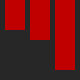Posted: June 7, 2011 in Silverlight
Tags: , , , , ,

Best animation performance in Silverlight 4 is obtained from the combination of procedural animation and a pixel shader, as reported in a previous blog. A pixel shader is not really meant to be used for spatial manipulation, but in Silverlight 4 vertex and geometry shaders are not available. Also, pixel shaders are limited to Model 2 shaders in Silverlight 4, and only limited data exchange is possible. The question is then, “how far can we push pixel shaders model 2”. Another previous blog post discussed a preliminary version of dispersion. This post is about Expansion. The effect is much like Dispersion, but the implementation is quite different – better if I may say so. Expansion means that a surface, in this case a playing video is divided up in blocks. These blocks then move toward, and seemingly beyond the edges of the surface.

The pixel shader was created using Shazzam. The first step is to reduce the image within its drawing surface, thus creating room for expansion. Expansion is in fact just translation of all the blocks in distinct direction that depend on the location of the block relative to the center of the reduced image.

In the pixel shader below, parameter S is for scaling, reducing, the pixel surface. Parameter N defines the number of blocks along an axis. So if N = 20, expansion concerns 400 blocks. In the demo App I’ve set the maximum for N to 32, which results in 1024 blocks tops. Parameter D defines the distance over which the reduced image is expanded. If the maximum value for D is the number of blocks, all the blocks (if N is even), or all but the center block (N is odd) just ‘move off’ the surface.

The code has been commented extensively, so should be self explanatory. The big picture is that the reduced, centered and then translated location of the blocks is calculated. Then we test whether a texel is in a bloc, not in an inter block gap. If the test is positive, we sample the unreduced, uncentered and untranslated image for a value to assign to the texel.

```sampler2D input : register(s0);

/// <summary>Reduces (S: Scales) the image</summary>
/// <minValue>0.01</minValue>
/// <maxValue>1.0</maxValue>
/// <defaultValue>0.3</defaultValue>
float S : register(C0);

/// <summary>Number of blocks along the X or Y axis</summary>
/// <minValue>1</minValue>
/// <maxValue>10</maxValue>
/// <defaultValue>5</defaultValue>
float N : register(C2);

/// <summary>Displaces (d) the coordinate of the reduced image along the X or Y axis</summary>
/// <minValue>0.0</minValue>
/// <maxValue>10.0</maxValue> // Max should be N
/// <defaultValue>0.2</defaultValue>
float D : register(C1);

float4 main(float2 uv : TEXCOORD) : COLOR
{
/* Helpers */
float4 Background = {0.937f, 0.937f, 0.937f, 1.0f};
// Length of the side of a block
float L = S / N;
// Total displacement within a Period, an inter block gap
float d = D / N;
// Period
float P = L + d;
// Offset. d is subtracted because a Period also holds a d
float o = (1.0f - S - D - d) / 2.0f;
// Minimum coord value
float Min = d + o;
// Maximum coord value
float Max = S + D + o;

// First filter out the texels that will not sample anyway
if (uv.x >= Min && uv.x <= Max && uv.y >= Min && uv.y <= Max)
{
// i is the index of the block a texel belongs to
float2 i = floor( float2( (Max - uv.x ) / P , (Max - uv.y ) / P  ));

// iM is a kind of macro, reduces calculations.
float2 iM = Max - i * P;
// if a texel is in a centered block,
// as opposed to a centered gap
if (uv.x >= (iM.x - L) && uv.x <= iM.x &&
uv.y >= (iM.y - L) && uv.y <= iM.y)
{
// sample the source, but first undo the translation
return tex2D(input, (uv - o - d * (N -i)) / S );
}
else
return Background;
}
else
return Background;
}
```

## Client Application

The above shader is used in an App that runs a video fragment, and can be explored at my App Shop. The application has controls for image size, number of blocks, and distance. The video used in the application is a fragment of “Big Buck Bunny”, an open source animated video, made using only open source software tools.

### Animation

Each of the above controls can be animated independently. Animation is implemented using Storyboards for the slider control values. Hence you‘ll see them slide during animation. The App is configured to take advantage of GPU acceleration, where possible. It really needs that for smooth animation. Also the maximum frame rate has been raised to 1000.

### Performance Statistics

The animations run at about 225 FPS on my pc. This requires significant effort as from the CPU –about 50% of the processor time. The required memory approaches 2.3Mb.1.Silverlight 5 – Moon boundary transition effects | Marc Drossaers says: